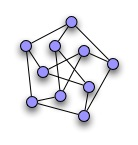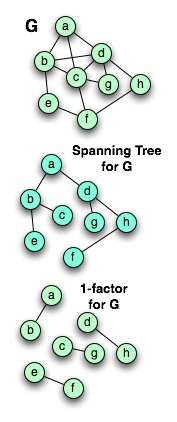# A Taxonomy of Some Basic Graphs

Naming Some Special Graphs
When we talk about graph theory – particularly when we get to some of the
interesting theorems – we end up referencing certain common graphs or type of graphs
by name. In my last post, I had to work in the definition of snark, and struggle around
to avoid mentioning another one, so it seems like as good a time as any to run through
some of the basics. This won’t be an exciting post, but you’ve got to do the definitions sometime. And there’s a bunch of pretty pictures, and even an interesting simple proof or two.

&uotA *complete* graph is a graph where every vertex has an edge to every other vertex.
We call a complete graph with N vertices KN. For example, the figure over to the right is K6. When a complete graph is a subgraph of a larger graph, it’s called a *clique*. If removing the clique makes a connected graph become disconnected, then it’s called a *clique separator*. (Clique separators are very useful in graph coloring.)If the vertices of a graph can be separated into two disjoint sets A and B such that every edge runs from a vertex A to a vertex in B, then we call it a *bipartite* graph. (Another way of stating this is that the graph can be 2-colored.) If there’s an edge from every node in A to every node in B, then we call it a *complete* *bipartite* graph. The complete bipartite graph between two sets A and B where A has N vertices, and B has M vertices, is
called KM,N. For example, over to the left is K4,3.
A bipartite graph
can *never* include a cycle whose length is odd. There’s a remarkably simple proof. In a bipartite graph, every path must alternate A to B to A to B. Any cycle must necessarily follow the pattern of an edge from A to B, followed by a path, followed by an edge from B to A. The path must also follow a pattern: B to A, subpath, A to B. There’s no way to add to the potential cyclic paths in a bipartite graph without adding *two* edges.
If a graph is connected and contains no cycles, then it’s called a *tree*. A disconnected
graph whose connected components are all trees is called a *forest*. You can easily prove that every tree is bipartite. Take a node, and call it the root. Take the nodes connected it, and call them its children, and call the root its parent. For each of those nodes, call the nodes connected to them *their* children, and so on. Now, starting at the root, color the root one color, the children the other, the grandchildren the first, great-grandchildren the second, and so on.A snark is, as I mentioned yesterday, a connected, bridgeless, cubic graph with chromatic index 4.
The graph over to the right is called *Petersen’s graph*. Every snark contains
Petersen’s graph as a subgraph.Given a graph G, a subgraph is called a *spanning subgraph* if it includes every vertex
in G. If G is connected, a connected spanning subgraph that is a tree is called a *spanning tree*. Every connected graph has a *minimum spanning tree* – that is, a spanning tree such that no spanning tree can contain fewer edges. A spanning subgraph of G whose nodes all have degree N is called an *N-factor* of G. To the left, there’s a graph G; a spanning tree, and a one-factor.
Given a graph G, if there is a cycle including every node, that’s called a *Hamiltonian cycle*. A graph can have multiple hamiltonian cycles.

## 0 thoughts on “A Taxonomy of Some Basic Graphs”

1.William

Okay, it may just be because it’s late and my brain is fried, but you’ve defined spanning tree and minimum spanning tree as if they’re two different things. However, I can’t think of a graph with a spanning tree that isn’t minimal. Am I missing something?

2.Googol

Does two vertex and one edge between can be seen as K1,1 a bipartite graph? If so, this bipartite graph only have one edge, odd number.

3.Googol

Oh, sorry, I miss that is a cycle edge number.

4.Anonymous

William is right: all spanning trees have the same number of edges. In fact, it’s trivial to show that a tree with N vertices has N-1 edges. “Minimum” spanning trees come in when edges are weighted, like lengths of paths from one hub to another.

5.Kerne

A spanning tree is any tree in a graph that includes all its nodes. Where each edge has a length (or “weight”), the weight of the tree is the summed weight of its edges.
If the graph is not already a tree, then it will have multiple different spanning trees. If the edges are not all of the same weight, then different spanning trees will have different weights. Then of all the possible spanning trees, the minimum spanning tree one with the lowest possible weight.

6.Anders

what program are you using for drawing all these wonderful graphs?

7.Alon A

More nit-picking: every snark contains Petersen’s graph as a *minor*, not as a sub-graph.
Petersen’s graph indeed has chromatic *index* 4, but yesterday you defined a snark as having chromatic *number* 4 🙂 Exercise: color Petersen’s graph with 3 colors.

8.Craig Helfgott

Oooh, please talk about Moore graphs! A while back I was fiddling with them, trying to find the one (probably non-existent) one with degree 57 and 3250 vertices. I’d managed to reduce the problem to finding a 56x56x56 Latin cube with some nice extra properties… at which point my head exploded.

9.Coin

The graph over to the right is called Petersen’s graph. Every snark contains Petersen’s graph as a subgraph.
…Huh. Why couldn’t then you replace that whole 500-page 4-coloring theorem with a proof that Petersen’s graph cannot be planar?

10.Coin

More nit-picking: every snark contains Petersen’s graph as a *minor*, not as a sub-graph.
Err, wait, I think that answers my question.

11.Alon A

Coin: Once you know that all snarks contain Petersen as a minor, you do indeed get 4CT since a graph which contains a non-planar graph as a minor cannot be planar itself.
However, the actual proof that all snarks have a Petersen minor is, in fact, more elaborate than the proof of 4CT 🙂

12.Coin

Ah. That’ll do it then.

13.Jonathan Vos Post

Will you be explaining random graphs, so important in network theories?
Recent example:
arXiv:0707.1786
Title: A new approach to the giant component problem
Authors: Svante Janson, Malwina Luczak
Subjects: Combinatorics (math.CO); Probability (math.PR)
We study the largest component of a random (multi)graph on n vertices with a given degree sequence. We let n tend to infinity. Then, under some regularity conditions on the degree sequences, we give conditions on the asymptotic shape of the degree sequence that imply that with high probability all the components are small, and other conditions that imply that with high probability there is a giant component and the sizes of its vertex and edge sets satisfy a law of large numbers; under suitable assumptions these are the only two possibilities. In particular, we recover the results by Molloy and Reed on the size of the largest component in a random graph with a given degree sequence.
We further obtain a new sharp result for the giant component just above the threshold, generalizing the case of G(n,p) with np=1+omega(n)n^{-1/3}, where omega(n) tends to infinity arbitrarily slowly.
Our method is based on the properties of empirical distributions of independent random variables, and leads to simple proofs.

14.Marion Delgado

I would like to add that K5 and K3,3 are special because a graph is planar (and things like the 4CT concern planar graphs) if and only if it does not have K5 or K3,3 as minors.
Also, both Petersen and Grötszch graphs (Grotzch is a 5-pointed-star drawn pentagram style with its middle pentagon’s points connected to a dot in the center) are triangle-free but not planar, which is rare. The Petersen has too many interesting properties to list, but one interesting thing about the Grotszch is that it’s 4-colorable and so is any graph of which it’s an induced subgraph (usually these are “dense triangle-free graphs”).

15.eyal229@gmail.com

Let T,T’ be two spanning trees of an undirected, connected, simple graph G with a weight function w on the edges.
Order the weights of edges in a monotone sequence:
T: a_1